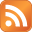# How to use Perl regular expression and save match to a new variable

Published on April 21st 2021 - Listed in Perl Coding

While working on a Perl script, I came across a problem when I wanted to save a part of a string into a new variable. In order to do so I decided to use a regular expression match and simply assign the match (group) to a new variable.

# At this point \$powerusage value is: "167 Watts"
my \$powerperf = \$powerusage =~ m/(\d+) [Ww]atts/i;
print "power usage \$powerusage (\$powerperf)\n";

Basically the \$powerusage variable is using a SimpleXML function to red a specific value from the XML document (saved as \$xml). This works fine and results in a string value of "167 Watts".

The \$powerperf variable should only contain the actual number of wattage, without any text. I tested the following regular expression m/(\d+) [Ww]atts/i on regex101 and everything seems correct:

However instead of the expected output "power usage 167 Watts (167)", \$powerperf always showed a "1" as value: "power usage 167 Watts (1)".

However when the regex is directly printed to the script's output, without saving the value to a variable, the output showed the correct value:

my \$powerperf = \$powerusage =~ m/(\d+) [Ww]atts/i;
print "power usage \$powerusage (\$powerperf)\n";
print "power usage in numbers:\n";
print \$powerusage =~ m/(\d+) [Ww]atts/i;
print "\n";

Output:

power usage 175 Watts (1)
power usage in numbers:
175

Research led to a question on stackoverflow where the same problem was discussed:

I get back a '1' as the value of \$link. I assume this is because it found '1' match. But how do I save the content of the match instead?

Luckily user ikegami gave a solution where the first match can be saved into a variable by putting the variable itself into parentheses.

Note the /g to get all matches. Those can't possibly be put into a scalar. You need an array. If you just want the first match [...] note the parens (and the lack of now-useless /g). You need them to call m// in list context.

By applying this knowledge, the declaration of \$powerperf was adjusted:

my (\$powerperf) = \$powerusage =~ m/(\d+) [Ww]atts/i;
print "power usage \$powerusage (\$powerperf)\n";

And the output is now correct:

power usage 158 Watts (158)